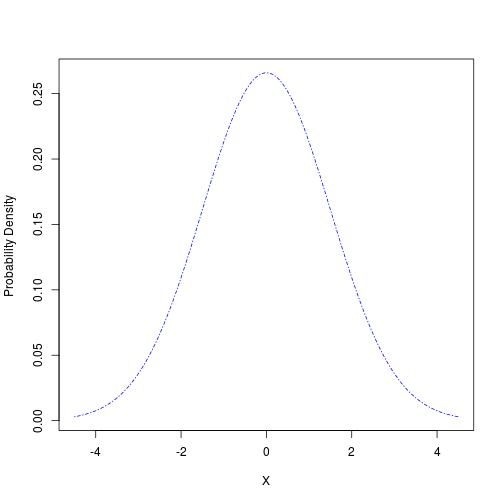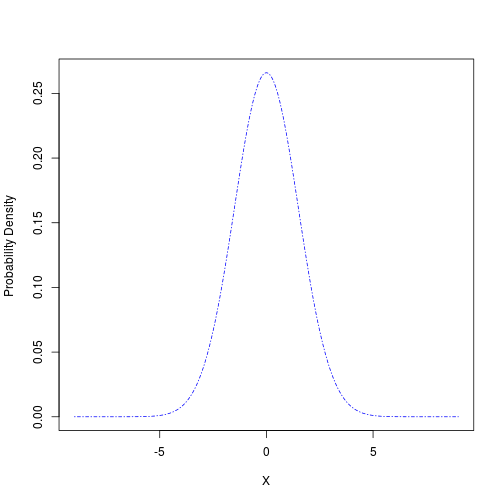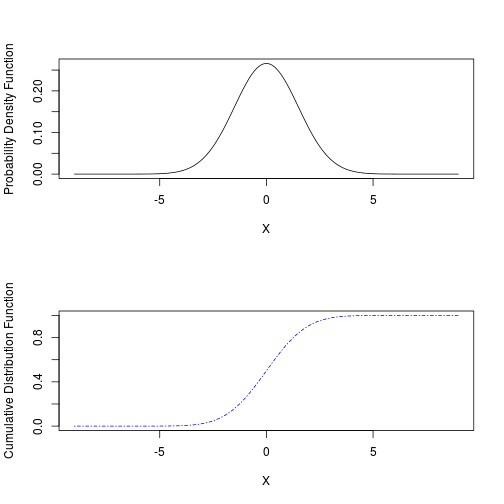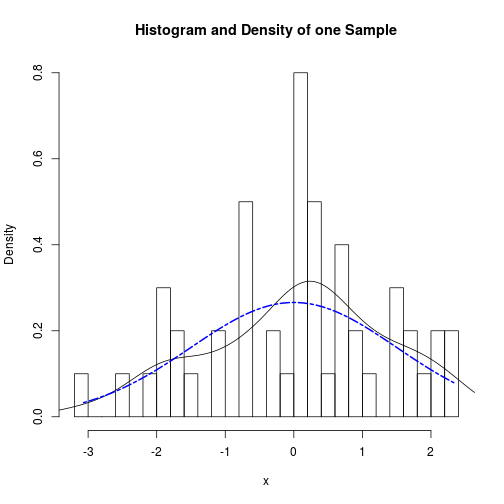### Filename: Normal1_2008.R
### Paul Johnson March 31, 2008
### This code should be available somewhere in http://pj.freefaculty.org.  If it is not
### email me <pauljohn@ku.edu>

mymean <- 0

mystd <- 1.5

myx <- seq( mymean - 3*mystd,  mymean+ 3*mystd, length.out=500)

myDensity <- dnorm(myx,mean=mymean,sd=mystd)

plot(myx, myDensity, type="n", xlab="X", ylab="Probability Density ")

lines(myx,myDensity,lty=4, col=4) ### change line type & color if you want#maybe broaden out x

myx <- seq( mymean - 6*mystd,  mymean+ 6*mystd, length.out=500)

myDensity <- dnorm(myx,mean=mymean,sd=mystd)
plot(myx, myDensity, type="n", xlab="X", ylab="Probability Density ")

lines(myx,myDensity,lty=4, col=4) ### change line type & color if you wantpar(mfcol=c(2,1))

plot(myx,  myDensity, type="n",  xlab="X",ylab="Probability Density Function")
lines(myx, myDensity )

myCumulProb <- pnorm(myx, mean=mymean, sd=mystd)
plot(myx, myCumulProb, type="n", xlab="X", ylab="Cumulative Distribution Function")
lines(myx,myCumulProb,lty=4, col=4) ### change line type & color if you wantpar(mfcol=c(1,1))

# What does one random sample from this distribution look like?

mySample <- rnorm(50, mean = mymean, sd = mystd)
hist(mySample, freq=FALSE, xlab="x", main="Histogram and Density of one Sample")hist(mySample, freq=FALSE, xlab="x", main="Histogram and Density of one Sample", breaks=20)

lines(density(mySample))# Compare against true probabilities
## 4 lines simply re-do previous 4, in case you closed the graph already.
mySample <- rnorm(50, mean = mymean, sd = mystd)
hist(mySample, freq=FALSE, xlab="x", main="Histogram and Density of one Sample", breaks=20)
lines(density(mySample))

xrange <- seq(min(mySample), max(mySample), by=0.1)
trueProbs <- dnorm(xrange,mean=mymean,sd=mystd)

lines( xrange, trueProbs, lty=6, col=4, lwd=2)hist (mySample, freq=F, xlab="X", main= paste("Normal Sample (50 Observations)\n Mean=", mymean, "Std.Dev=", mystd))t1 <-  bquote( mu== .(mymean))

t2 <-  bquote( sigma== .(mystd))

hist (mySample, freq=F, xlab="X", main= t1 )
mtext(t2, 3)